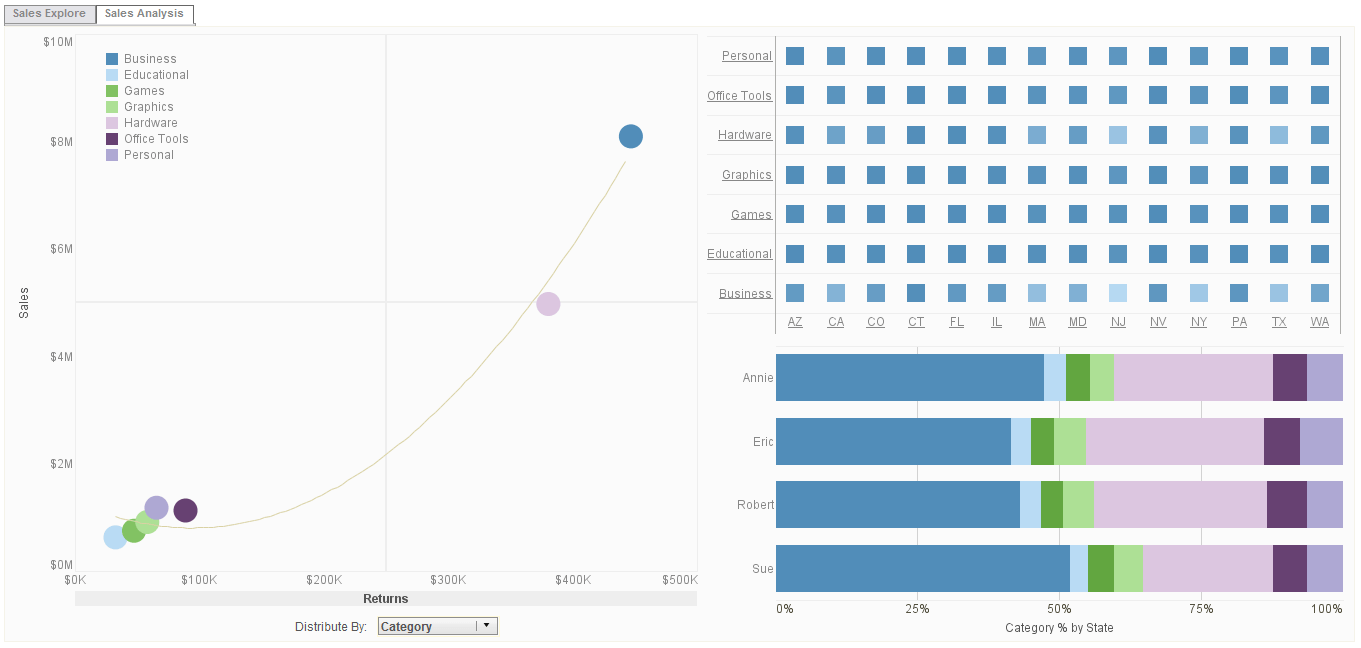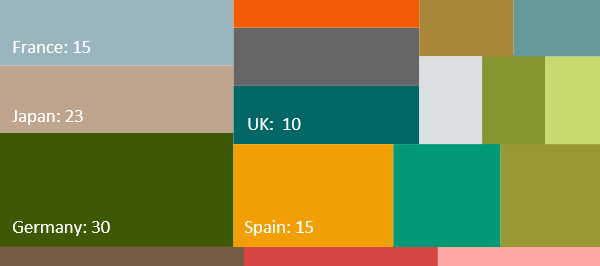# InetSoft Reporting Software: Report Script

Report script that modifies 'graph' should be placed at the element level. See Adding Element-Level Script.Click this screenshot to view a three-minute demo and get an overview of what InetSoft’s BI dashboard reporting software, Style Intelligence, can do and how easy it is to use.

## DataSet.getRowCount()

Returns the number of rows in the DataSet (including the header row).

Example:

```importPackage(inetsoft.graph)
importPackage(inetsoft.graph.data)
importPackage(inetsoft.graph.element)
importPackage(inetsoft.graph.aesthetic)
importPackage(inetsoft.graph.guide.form)

// Create a chart with two datasets:
var arr = [["State", "Total", "Profit"],
["NJ", 200, 25],
["NY", 300, 150]];
dataset = new DefaultDataSet(arr);
graph = new EGraph();
var elem1 = new IntervalElement("State", "Total");
var elem2 = new IntervalElement("State", "Profit");
var frame = new StaticColorFrame(java.awt.Color.red);
elem2.setColorFrame(frame);

// Loop through the rows of the second dataset (Profit),
// and place labels on the bars.
for (var i=0; i}```View live interactive examples in InetSoft's dashboard and visualization gallery.

## DataSet.getColCount()

Returns the number of columns in the DataSet.

Example (Report or Viewsheet):

```importPackage(inetsoft.graph)
importPackage(inetsoft.graph.data)
importPackage(inetsoft.graph.element)
importPackage(inetsoft.graph.aesthetic)
importPackage(inetsoft.graph.guide.form)

// Create a chart with two datasets:
var arr = [["State", "Total", "Profit"],
["NJ", 200, 25],
["NY", 300, 150]];
dataset = new DefaultDataSet(arr);
graph = new EGraph();
var elem1 = new IntervalElement("State", "Total");
var elem2 = new IntervalElement("State", "Profit");
var frame = new StaticColorFrame(java.awt.Color.red);
elem2.setColorFrame(frame);

// Loop through the rows and columns,
// and place labels on the bars.
for (var i=0; i```

## DataSet.setOrder(dim,arr)

Set a manual label ordering for a given dimension field.

Parameters

```dim String giving name of the dimension to sort
arr Array of strings with desired label order:
['label1','label2','label3',...]```
 #1 Ranking: Read how InetSoft was rated #1 for user adoption in G2's user survey-based index

Example (Report or Viewsheet):

```importPackage(inetsoft.graph)
importPackage(inetsoft.graph.data)
importPackage(inetsoft.graph.element)
importPackage(inetsoft.graph.aesthetic)
importPackage(inetsoft.graph.guide.form)
var arr = [["State", "Quantity"], ["NJ", 200],
["NY", 300], ["PA", 25]];
dataset = new DefaultDataSet(arr);
dataset.setOrder('State',['PA','NY','NJ']);
graph = new EGraph();
var elem = new IntervalElement("State", "Quantity");